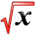# Mean Value TheoremThis article is part of the MathHelp Tutoring Wiki

Let f(x)= sqrt(2x-x2) on the interval [0,2]. Find all numbers c, 0<c<2, which satisfy the conclusion of the mean value theorem.

f(x)= sqrt(2x-x2)

f'(x)=(1-x)/sqrt(2x-x2)

f(x) is differential and continous on (0,2),

Thus f'(c)= (f(2)-f(0))/(2-0)

f'(c)=(1-c)/sqrt(2c-c2)=(0-0)/2=0

(1-c)=0 => c=1